# Change of Phase/State (Phase Transition) with Examples

Change of Phase

Matters can be in four states like solid, liquid, gas and plasma. Distance between the molecules or atoms of the matter shows its state or phase . Temperature and pressure are the only factors that affect the phases of matter. Under constant pressure, when you heat matter, its speed of motion increases and as a result the distance between the atoms or molecules becomes larger. If you give heat to a solid substance, its temperature increases up to a specific point and after this point temperature of it is constant and it starts to change its phase from solid to liquid. Another example that all you in experience daily life, when you heat water it boils and if you continue to give heat it starts to evaporate. In this section we will learn these changes in the phases of substances and learn how to calculate necessary heat to change the states of them.

Melting and Freezing

If solid matters gain enough heat they change state solid to liquid. Heat is a form of energy and in this situation it is used for the break the bonds of the atoms and molecules. Heated atoms and molecules vibrate more quickly and break their bonds. We call this process melting changing state solid to liquid. Inverse of melting is called freezing, changing state liquid to solid, in which atoms and molecules lost heat and come together, their motion slows down and distance between them decreases.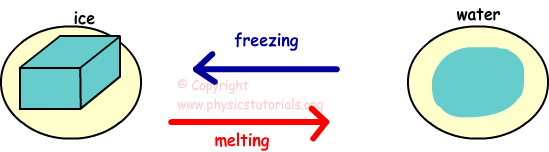Look at the given graph which shows the melting of the ice.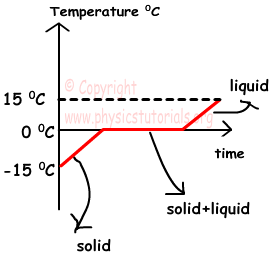This is a phase of change of water from solid to liquid. As you can see at the beginning ice is at -15 ºC, we give heat and its temperature becomes 0 ºC which is the melting point of ice. During melting process temperature of the ice-water mixture does not change. After all the mass of ice is melted its temperature starts to rise.

Every solid matter has its own melting point; we can say that melting point is a distinguishing property of solids. Inverse of this process is called freezing in which liquid lost heat and change phase liquid to solid. Freezing point and melting point are the same for same matter and it is also distinguishing property of matter.

We find the heat necessary for melting the solid substance with following formula;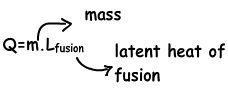Lfusion, like specific heat, it shows how much heat you should give for melting/freezing unit of mass. For example, 3,3×105 joule/kg is the latent heat of fusion for ice and liquid.

Example: Find the amount of heat for melting the ice having mass 1,3kg at -10 ºC? (Lfusion =3, 3×105 joule/kg cice=2,2X103j/kg.ºC)

We first increase the temperature of the ice from -10 ºC to 0 ºC (melting point).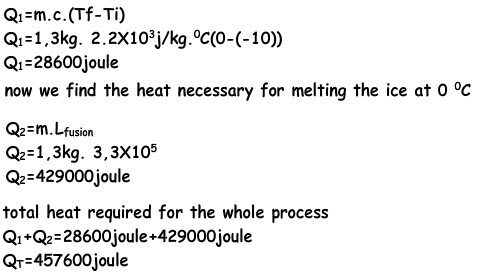Effects of Pressure and Impurity on Freezing and Melting Point

Pressure is the force exerting on the surface perpendicularly. Thus, it helps to keep particles together. If volume of the matter increases after melting, pressure decreases the melting point. On the contrary, if the volume of the substance decreases after melting, pressure increases the melting point of the matter. For example, when you walk on the snowy road you observe that snow under your feet melt later than around, because you exert pressure on it with your feet. Ice melting at 0 ºC can be melt at -3 ºC with the applied pressure on it. Impurity like pressure affects the latent heat of fusion. For instance, salty water freezes under 0 ºC.

Boiling Evaporation and Condensation

Evaporation is the change of phase from liquid to gas. Evaporation occurs only at the surface of the water and at every temperature. However, evaporation is directly proportional to the temperature, increasing in the temperature increasing in the rate of evaporation. Inverse of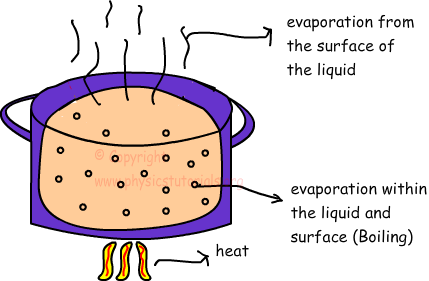this process is called condensation in which; gas molecules/atoms lost heat and change phase from gas to liquid. As in the case of melting, when you give heat to liquid, at one certain point its temperature does not change. Gained heat spent on breaking the bonds between molecules and atoms. At this temperature, vapor pressure of the liquid is equal to the pressure of surrounding. During this process evaporation occurs in everywhere of the liquid which is called boiling. Boiling point is a distinguishing property of liquids; each matter has its own boiling point. For example, water boils at 100 ºC in atmospheric pressure. We use the following formula to find required heat to boil liquid matter.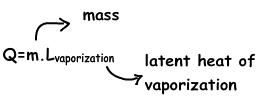Where; m is the mass of the liquid matter and Lvaporization is the latent heat of vaporization that shows the necessary heat to evaporate unit of mass. For example, you should give 2,3X106 joule heat to change the phase of water from liquid to gas.

Example: Find the amount of heat for evaporating 2,8kg of water at 45 Cº? (Lvaporization =2, 3×106 joule/kg cwater=4190j/kg.Cº)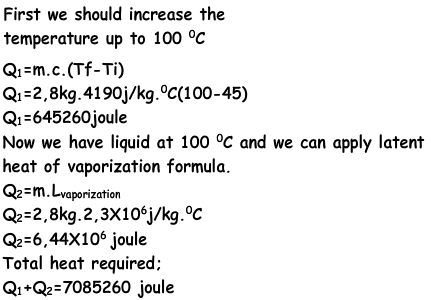Effects of Pressure and Impurity on Boiling Point

Boiling occurs only when the vapor pressure of liquid and pressure of outside equals to each other. If the pressure of outside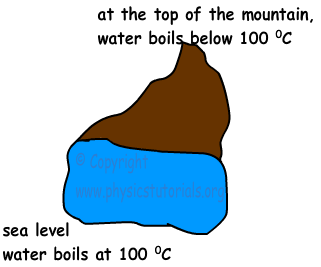increases then the boiling point of the liquid also increases. On the contrary, if the pressure of the outside decreases, then boiling point of the liquid also decreases. For example, at the top of a mountain atmospheric pressure is lower than the atmospheric pressure of the sea level. In addition to this, impurity of the liquid matter also affects the boiling point of that matter. For instance, if you mix water with a salt or sugar, you increase the boiling point of the water

Sublimation

Sublimation is the change of state from solid to gas. Some of the solid matters change their states directly to the gas with the gained heat. For example, dry ice (frozen CO2) sublimate when heat is given. Inverse of this process is called deposition, in which gas matters lost heat and change their phase to solid.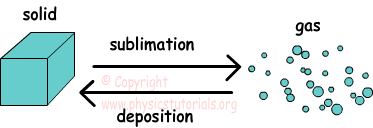Example: Graph given below shows the relation of temperature and gained heat on different matters. Which ones of them are possible?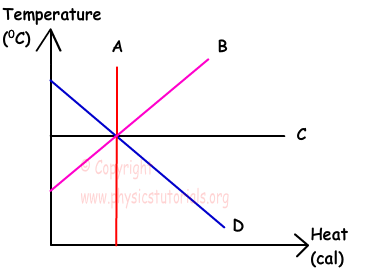Line of A shows the relation that, gained heat by the matter is constant however, its temperature is increasing. Such a relation between heat and temperature of the matter is not possible.

Line B shows that, temperature of the substance increases with the gained heat. It is possible.

Line C shows that, matter gains heat but its temperature stays constant. This is also possible; C can be change of its phase.

Line D says that, matter gains heat however, its temperature decreases. This situation is not possible.

Heat Temperature and Thermal Expansion Exams and Solutions

Related